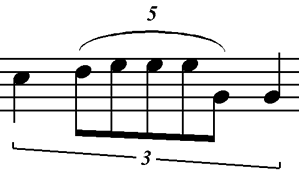Documentation
Alphabetic list of elements (v1.0)

 Duration and tuplet_ratio


Element duration defines rhythmical values, by using a fraction notation. The value encoded is the one appearing in the score, without considering additional information such as augmentation dots or ties.

Attributes

• num, a mandatory attribute that defines the numerator of the fraction.
• den, a mandatory attribute that defines the denominator of the fraction.

Element tuplet_ratio defines rhythmic values of notes within tuplets. However, the graphical rhythmic value is encoded in the previous duration element (e.g., for a triplet of eigths, each eigths has <duration num="1" den="8" />). On the other hand, the meaning of this sub-element corresponds to the number of tuplet subdivisions in a given number of real subdivisions, according to the sentence "enter x values in y values" (e.g., for a triplet of eighths that takes the place of two eighths, each eighth has <tuplet_ratio enter_num="3" enter_den="8" in_num="2" in_den="8" />).

Attributes

• enter_num, a mandatory attribute that defines the numerator of the fraction representing the number of tuplet values.
• enter_den, a mandatory attribute that defines the denominator of the fraction representing the number of tuplet values.
• enter_dots, an optional attribute to support dotted values in tuplet values.
• in_num, a mandatory attribute that defines the numerator of the fraction representing the number of actual values in the current time signature.
• in_den, a mandatory attribute that defines the denominator of the fraction representing the number of actual values in the current time signature.
• in_dots, an optional attribute to support dotted values in actual values.

Examples...
 Laboratorio di Informatica Musicale Dipartimento di Informatica Università degli Studi di Milano Via G. Celoria, 18 - 20133 Milano, ITALYGoffredo Haus, PAR1599 chair Phone: +39 02 50316 222 E-mail: goffredo.haus@unimi.it Luca A. Ludovico, PAR1599 vice-chair Phone: +39 02 50316 382 E-mail: luca.ludovico@unimi.it Laboratory Phone: +39 02 50316 382 E-mail: lim@di.unimi.it